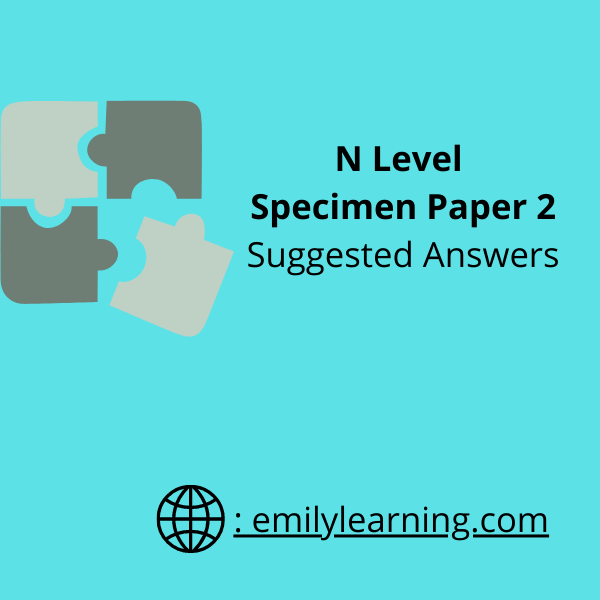# Suggested Answer for N Level A-Math Specimen Paper 2Here you will find the answers and worked solutions for N Level A-Math Specimen Paper 2. You can find specimen paper 2 on the Singapore Examinations and Assessment Board (SEAB) here.

Click on the question below to go straight to the worked solutions for that question:

Question 1

Question 2

Question 3

Question 4

Question 5

Question 6

Question 7

Question 8

Question 9

Question 10

## N Level Specimen Paper 2 Question 1 on Polynomial

Students are tested on the concept of identities in the chapter on polynomials. An alternative method to solve this question is by long division.

## N Level Specimen Paper 2 Question 2 on Rate of Change (Application of Differentiation)

In this question, students are expected use chain rule to solve a question involving rate of change.

## N Level Specimen Paper 2 Question 3 on Quadratic functions

In this question, students are expected to complete the square of a quadratic function. Subsequently, use the complete the square form to find the minimum point.

## N Level Specimen Paper 2 Question 4 on Differentiation (using differentiation to find equation of tangent)

In this question, students need to find the equation of a tangent. They’ll need to find the gradient of the tangent first by differentiation, before proceeding to find equation of tangent.

## N Level Specimen Paper 2 Question 5 on Factor and Remainder Theorem

This is a factor and remainder theorem question. Students also need to solve simultaneous equations as part of the steps to solve for the unknowns (a and b).

## N Level Specimen Paper 2 Question 6 on Differentiation

This is a straight forward question on differentiation techniques. Students are expected to use the product rule and/ or quotient rule. Note that for part (a), you can also solve using the product rule.

## N Level Specimen Paper 2 Question 7 on Integration, finding area between two graphs

In this question, students are expected to find the area between two graphs using integration.

## N Level Specimen Paper 2 Question 8 on partial fractions and differentiation

This is a question that tests quite a number of concepts: partial fractions for part a, differentiation for par b and inequalities in part c.

## N Level Specimen Paper 2 Question 9 on Trigonometry R- formula

A question on the use of R- formula, and then, solving an equation.

## N Level Specimen Paper 2 Question 10 on Coordinate Geometry for circles

The last question is on the coordinate geometry, in particular, that involving circles for part a. For part b, it’s essentially solving simultaneous equation (non- linear one) to find the points of intersection and then mid point, and in part c, use the formula to find distance between two points.

## Learn On-demand – A- Math Courses

If you want to learn a particular topic in detail, check out our on-demand O Level A Math courses here.

Suggested Answers for other A Math Papers: